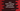# Java Math copySign method explanation with examples## Java Math copySign method explanation with examples:

The copySign method is a small utility method defined in the Math class. This method is used to copy the sign of one parameter to another parameter. In this post, we will learn how copySign method works with its definition and examples with different values.

### Definition of copySign:

The definition of copySign is defined as below:

``public static double copySign(double magnitude, double sign)``

and

``public static float copySign(float magnitude, float sign)``

This is a static method defined in the Math class. We can call this method directly without creating any object of this class.

We can pass two double or float values to this methods. This methods returns either double or float values.

It returns the first floating-point argument with the sign of the second floating-point argument. The first parameter is the magnitude of the result and the second parameter is the sign to use in the result.

#### Return value of copySign:

This method returns a value with magnitude as the magnitude and it takes the sign from the second parameter sign.

### Example with floating-point parameters:

Let’s try copySign with two floating-point values as the parameters:

``````class Main {
public static void main(String[] args) {
float firstValue = 10.3f;
float secondValue1 = 12.4f;
float secondValue2 = -13.22f;

System.out.println(Math.copySign(firstValue, secondValue1));
System.out.println(Math.copySign(firstValue, secondValue2));
}
}``````

Here, three different floating-point variables are initialized with different values. We are calling the copySign method with different values.

If you run this program, it will print:

``````10.3
-10.3``````

For the first one, it is printing the firstValue and it takes the sign from the secondValue1. Similarly, for the second one, it picks the sign from secondValue2.

### Example with double parameters:

Let’s try this example again with double values as the parameters:

``````class Main {
public static void main(String[] args) {
double firstValue = -12.3;
double secondValue1 = 12.4;
double secondValue2 = -13.22;

System.out.println(Math.copySign(firstValue, secondValue1));
System.out.println(Math.copySign(firstValue, secondValue2));
}
}``````

The parameters are changed to double in this example. It will print:

``````12.3
-12.3``````

It picks the sign from the second argument.

### Conclusion:

In this post, we learned how to use the copySign method with two different examples with floating point and double parameters. This is an useful utility method of Java Math class to copy the sign of one value to another.